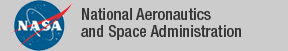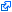### Top File of PVS measure_integration Library

```
%------------------------------------------------------------------------------
% Top file for measure_integration_fnd
%
%     Author: David Lester, Manchester University, NIA, Université Perpignan
%
% All references are to SK Berberian "Fundamentals of Real Analysis",
% Springer, 1991
%
%     Version 1.0            1/5/07   Initial Version
%------------------------------------------------------------------------------

top: THEORY

BEGIN

IMPORTING

% Local Extras
partitions,              % Partitioning a set into a setofsets
pointwise_convergence,   % Pointwise functional convergence
sup_norm,                % sup_norm(f) = sup(abs(f))
product_sections,        % sections of sets [T1,T2]

% Borel sets and functions
subset_algebra_def,      % Definitions of Sigma/Subset Algebras
subset_algebra,          % Properties of Subset Algebras
sigma_algebra,           % Properties of Sigma Algebras
product_sigma_def,       % Product sigma-algebra definition
product_sigma,           % product sigma-algebras properties
borel,                   % Borel sets
hausdorff_borel,         % In Hausdorff Space singletons are Borel
borel_functions,         % Borel Functions
identity_borel,          % ... preserved by identity and ...
composition_borel,       % ... and composition
real_borel,              % Borel sets of the reals

% Measures
generalized_measure_def, % Measures over setofsets containing emptyset
measure_def,             % Measures over a _subset_ algebra
measure_space_def,       % Measurable sets and functions on a sigma-algebra
measure_space,           % Simple functions and their limits
outer_measure_def,       % Outer measure definition
ast_def,                 % Generalized Measure-induced outer measures
outer_measure,           %  ... as above on subset algebras
outer_measure_props,     % outer measure properties
measure_props,           % measure properties
measure_theory,          % negligible/null definitions; also ae properties
monotone_classes,        % Monotone Class Lemma
hahn_kolmogorov,         % Hahn-Kolmogorov extension theorem

% Finite Measures
finite_measure,

% Extras for complete measures
complete_measure_theory, % Extras for complete measures
measure_completion_aux,  % The gory details for ...
measure_completion,      % Completing the measure, by making all
%    negligible sets null

% Integration
isf,                     % integrable simple functions
nn_integral,             % integrals of functions [T->nnreal]
integral,                % integrals of functions [T->real]
finite_integral,         % integrals over finite measures
integral_convergence_scaf, % Preliminary for Monotone Convergence theorem
integral_convergence,    % Monotone and dominated convergence theorems
complete_integral,       % Simplified form of above for complete measures
indefinite_integral,     % Indefinite integrals
measure_equality,        % Integrals on equal measures
measure_contraction,     % Measures on subsets of T ...
measure_contraction_props,  % ... and integrability
sigma_finite_measure_props, % specialization for sigma-finite measures

% Products
product_finite_measure,  % Products of finite measures
product_measure,         % Products of sigma-finite measures

% Product Integrals
product_integral_def,    % Product integral defs
finite_fubini_scaf,      % Fubini-Tonelli Lemmas for finite measures and
%   integrable simple functions
finite_fubini_tonelli,   % Fubini-Tonelli Lemmas for finite measures
finite_fubini,           % Fubini's Theorem for finite measures
fubini_tonelli_scaf,     % Preparatory results for ...
fubini_tonelli,          % Fubini-Tonelli Lemmas for sigma-finite measures
fubini                   % Fubini's Theorem for sigma-finite measures

END top
```
The tagidentifies links that are outside the NASA domain